# question 1 please ame: Last four digits of student ID: now all your work leading up...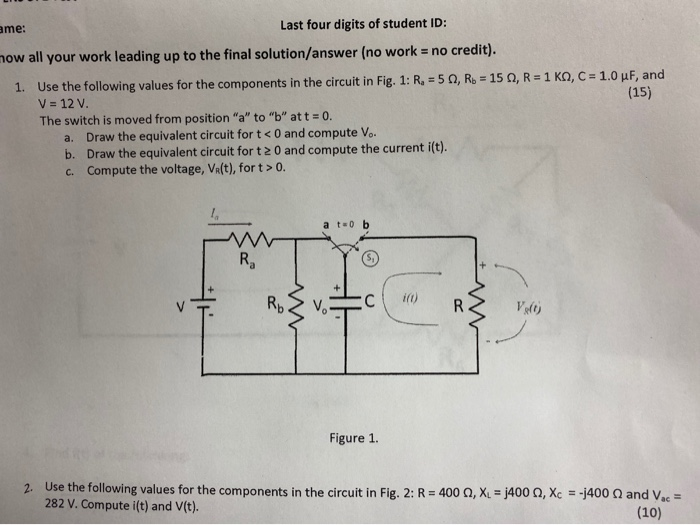ame: Last four digits of student ID: now all your work leading up to the final solution/answer (no work = no credit). 1. Use the following values for the components in the circuit in Fig. 1: R = 5, R. = 150, R = 1 K, C = 1.0 uF, and V = 12 V. (15) The switch is moved from position "a" to "b" att = 0. a. Draw the equivalent circuit for t < 0 and compute V.. b. Draw the equivalent circuit fort 20 and compute the current i(t). C. Compute the voltage, Va(t), fort > 0. a t=0 b Ro 2 c. RR van Figure 1. 2. Use the following values for the components in the circuit in Fig. 2: R=400 , XL = 400, Xc = -2400 282 V. Compute i(t) and V(t). and Vac (10)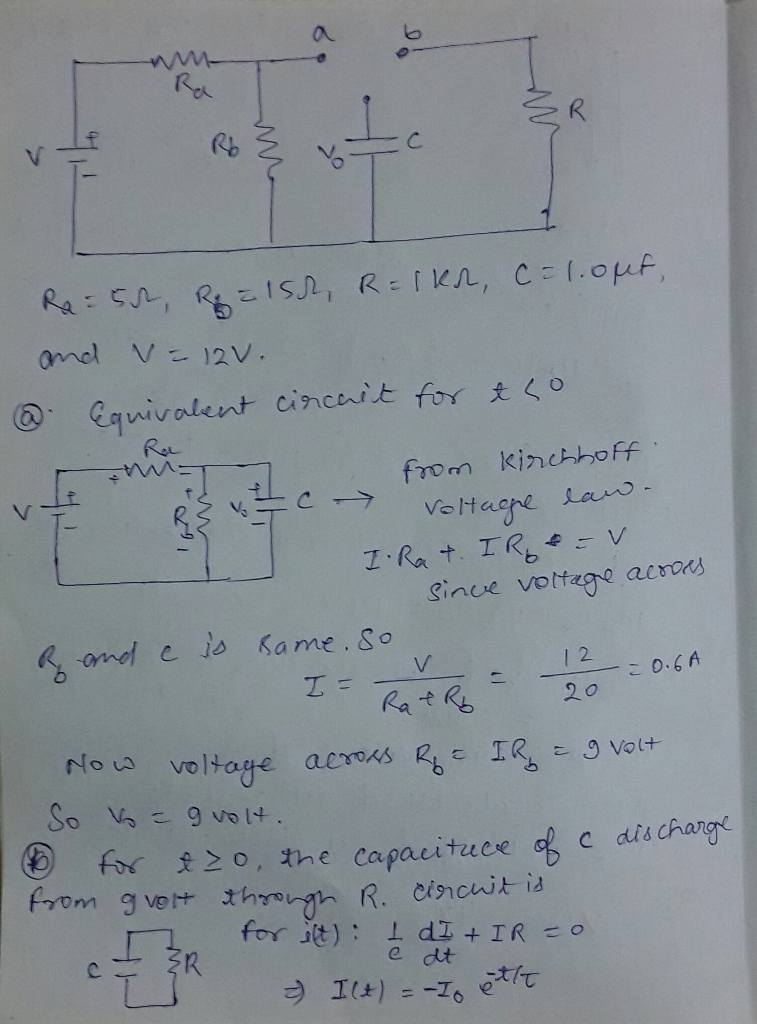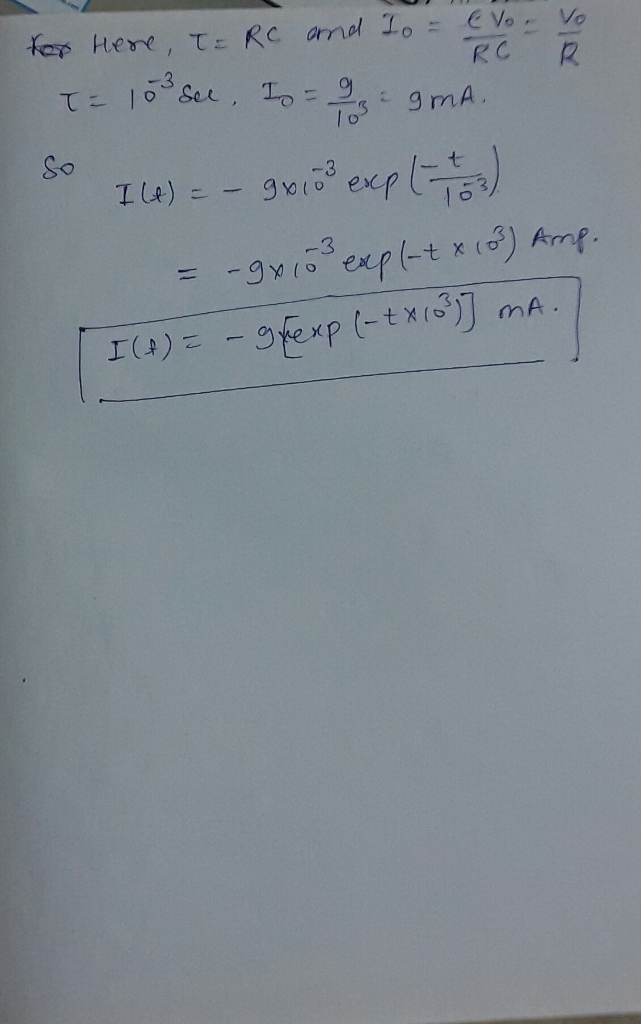#### Earn Coin

Coins can be redeemed for fabulous gifts.

Similar Homework Help Questions
• ### Show all your work leading up to the final solution/answer (no work no credit). 1.0 pP,...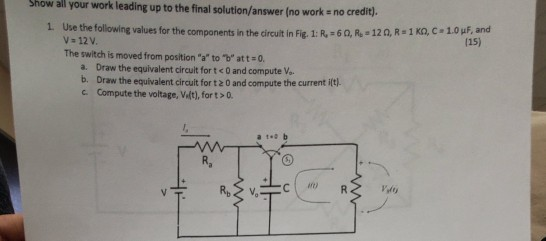Show all your work leading up to the final solution/answer (no work no credit). 1.0 pP, and (15) 0Se the following values for the components in the circult in Fig. 1: R, 60, R - 120, R 1 KO, C V 12V The switch is moved from position "a" to "b" at t 0. a. Draw the equivalent circuit fort<0 and compute Vo b. Draw the equivalent circult for t 2 0 and compute the current i(t) c Compute the...

• ### just do question 26 and 30 and show all your work (a) Select R so that 26. For the cincuit of Fig. 943,.40 30u-) m v...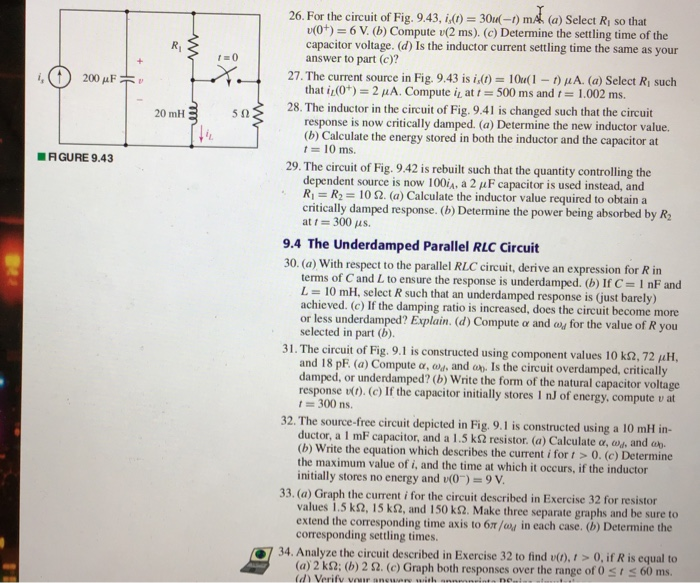just do question 26 and 30 and show all your work (a) Select R so that 26. For the cincuit of Fig. 943,.40 30u-) m v(0+) 6 V. (b) Compute (2 ms). (c) Determine the settling time of the capacitor voltage. (d) Is the inductor current settling time the same as your answer to part (c)? 27. The current source in Fig. 9.43 is id) = 101(1-1) μ A. (a) Select Ri such that iLO")-2 μΑ. Compute L at t-500...

• ### Please show all work The de-de converter shown in Fig. 1 operates in continuous conduction mode....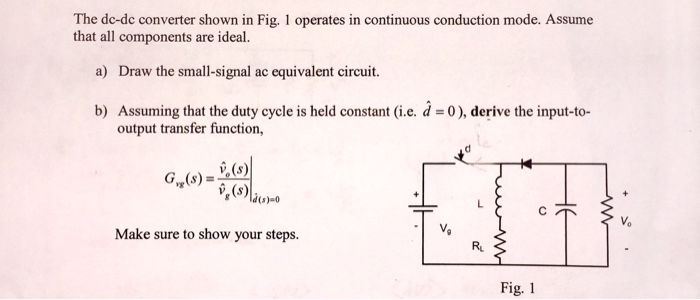Please show all work The de-de converter shown in Fig. 1 operates in continuous conduction mode. Assume that all components are ideal. a) Draw the small-signal ac equivalent circuit. b) Assuming that the duty cycle is held constant (i.e. d-0), derive the input-to- output transfer function v (s) 1) Make sure to show your steps. Ru Fig. 1

• ### Engineering circuit analysis by Hayt 8th edition question 27 and figure 9.43 I think 10u(1-t) means 10 (for t<1)...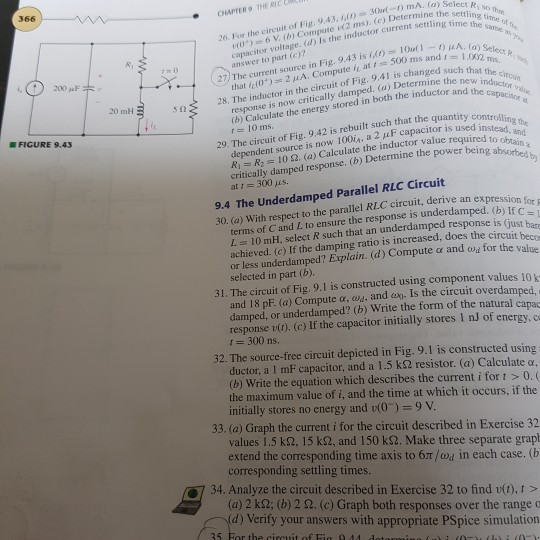Engineering circuit analysis by Hayt 8th edition question 27 and figure 9.43 I think 10u(1-t) means 10 (for t<1) and 0 (for t>1) then, I can't remove this current source because it continuously make 10micro A (at t=500ms, t=1.002ms) I don't know what's wrong now.. 366 26. For the circuit of Fig. 9,43, 1 30-) mA. (a) Select R, so th O)6 V (b) Compute e2 ms). (c) Determine the settling, time of t capacitor voltage. (d) Is the inductor...

• ### Please do not copy the previous answer for this question. and please explain and write clearly....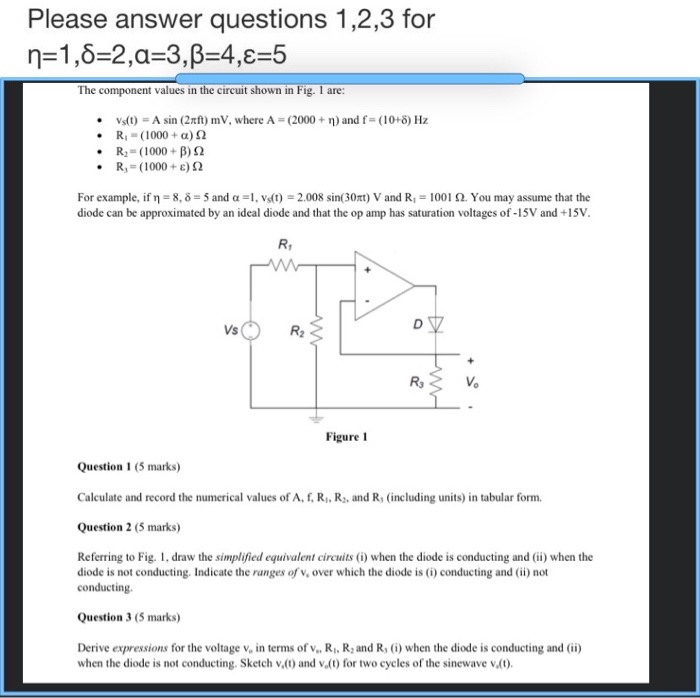Please do not copy the previous answer for this question. and please explain and write clearly. Many thanks! Please answer questions 1,2,3 for n=1,8=2,a=3,B=4,8=5 The component values in the circuit shown in Fig. I are: • • • • vs(t) = A sin (2 reft) mV, where A = (2000+ n) and f = (10+8) Hz Ri -(1000 + a)2 R2-(1000+B) 22 R, (1000+ €) 22 For example, if n = 8,8 = 5 and a=1, Vs(t) = 2.008 sin(...

• ### please fully explain all parts Question 1: Answer all eight parts of Question 1. All questions...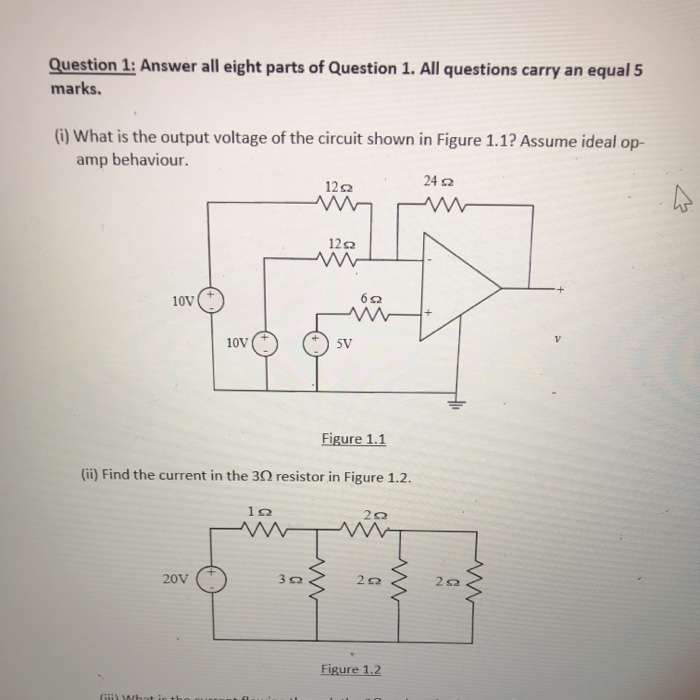please fully explain all parts Question 1: Answer all eight parts of Question 1. All questions carry an equal 5 marks. (i) What is the output voltage of the circuit shown in Figure 1.1? Assume ideal op- amp behaviour. 24 22 min m 10v lov o su Figure 1.1 (ii) Find the current in the 3 resistor in Figure 1.2. 20v – 30 20 Figure 1.2 - + ~ Fit to page Page view (ii) What is the current flowing...

• ### PLEASE SHOW ALL WORK AND TO USE PSPICE AND FOLLOW ALL DIRECTIONS. STUDENT ID=378564 Assignment: 1....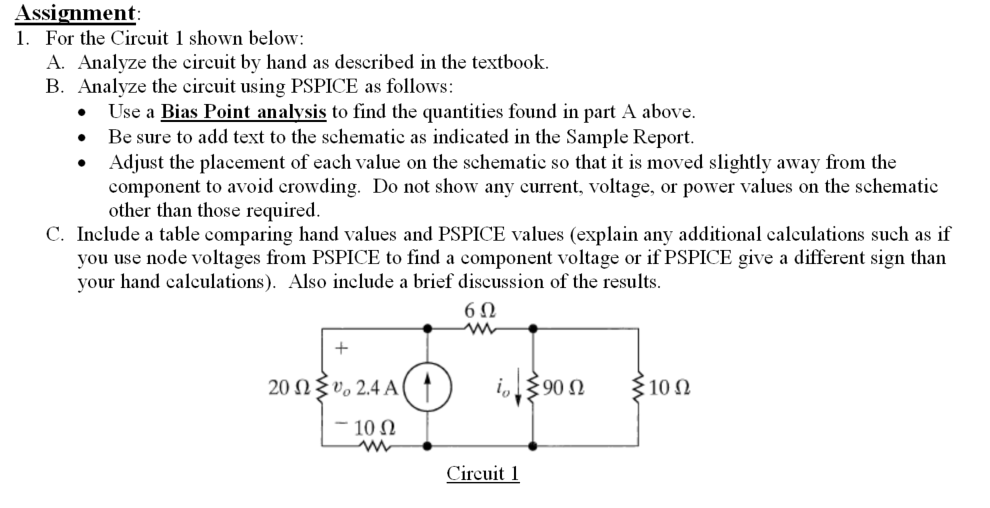PLEASE SHOW ALL WORK AND TO USE PSPICE AND FOLLOW ALL DIRECTIONS. STUDENT ID=378564 Assignment: 1. For the Circuit 1 shown below: A. Analyze the circuit by hand as described in the textbook. B. Analyze the circuit using PSPICE as follows: Use a Bias Point analysis to find the quantities found in part A above. Be sure to add text to the schematic as indicated in the Sample Report. Adjust the placement of each value on the schematic so that...

• ### ANSWER ALL PLEASE Question 43 2 pts The rms voltage across and the rms current through...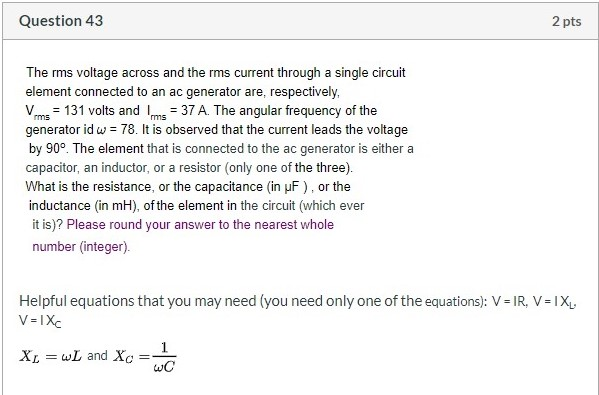ANSWER ALL PLEASE Question 43 2 pts The rms voltage across and the rms current through a single circuit element connected to an ac generator are, respectively, Vrms = 131 volts and Ims = 37 A. The angular frequency of the generator id w = 78. It is observed that the current leads the voltage by 90°. The element that is connected to the ac generator is either a capacitor, an inductor, or a resistor (only one of the three)....

• ### I NEED HELP WITH QUESTION #5 PLEASE AND CAN SOME FILL OUT THE FIRST ROW OF...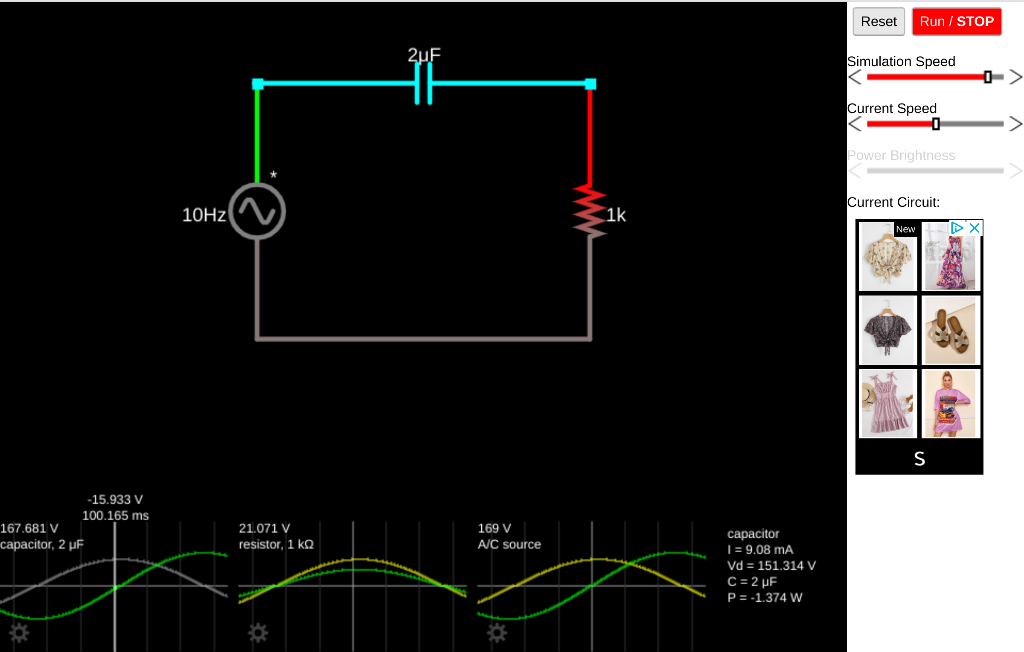I NEED HELP WITH QUESTION #5 PLEASE AND CAN SOME FILL OUT THE FIRST ROW OF MY TABLE FOR 10Hz. I DONT KNOW HOW TO DO IT SO IF SOME CAN FILL OUT THE FIRST TABLE AND SHOW ME HOW, THEN I CAN FINISH THE REST. THANK YOU. Reset Run / STOP 2uF Simulation Speed Current Speed 0 Power Brightness Current Circuit: 10Hz 1k New X S -15.933 V 100.165 ms 167.681 V capacitor, 2 UF 21.071 V resistor, 1...

• ### ANSWER ALL PLEASE Question 35 2 pts A charged particle (with q = 1.0 x 10-2...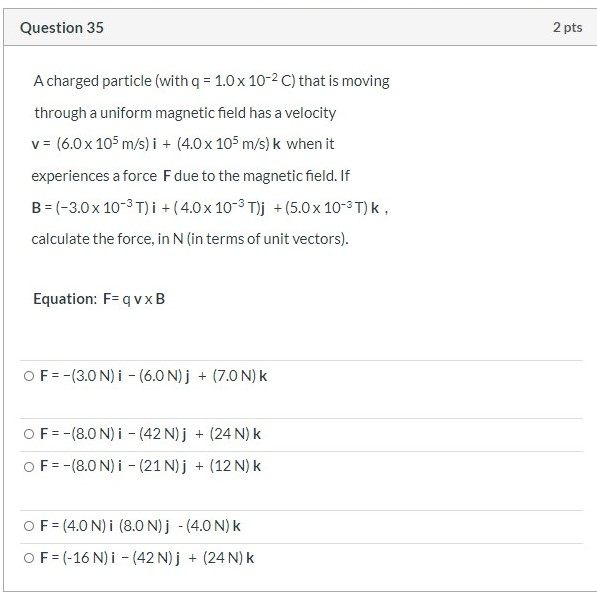ANSWER ALL PLEASE Question 35 2 pts A charged particle (with q = 1.0 x 10-2 C) that is moving through a uniform magnetic field has a velocity v = (6.0 x 105m/s) i + (4.0 x 105m/s) k when it experiences a force F due to the magnetic field. If B = (-3.0 x 10-3T)i + (4.0 x 10-3T)j + (5.0 x 10-3T)k, calculate the force, in N (in terms of unit vectors). Equation: F=qvxB OF=-(3.0 N)i - (6.0N)j...